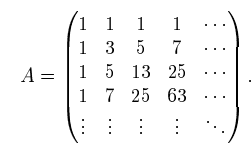# How to find the determinant of this matrix?

LCSphysicist
Homework Statement:
.
Relevant Equations:
.I think you all can see that ##a_{(i+1,j+1)} = a_{i,j} + a_{i+1,j} + a_{i,j+1}##

Now the determinant always give me problem. I have and idea to reduce this matrix by Chio to a 2x2 matrix and find the determinant of this 2x2.

Put i was not able to see any pattern to find what how the 2x2 matrix would be (beside symmetric)

Any tips?

Last edited by a moderator:
•etotheipi

Mentor
2022 Award
I would first try to write the matrix as a product of two simpler matrices, because the construction rule is similar to matrix multiplication. If this would be too complicated, I'd try the polynomial method: ##\det = \sum_{\sigma\in S_n}(-1)^n \ldots##

docnet
I want to suggest using row operations to reduce the matrix to something more manageable.

What could be helpful is the following.

Adding or subtracting any two rows of a matrix does not change the determinant.
Exchanging two rows of a matrix changes the sign of the determinant.

Mentor
2022 Award
Another idea is to prove by induction that the determinant equals ##2^{(n^2-n)/2}=2^{\binom n 2}##.

Last edited:
•